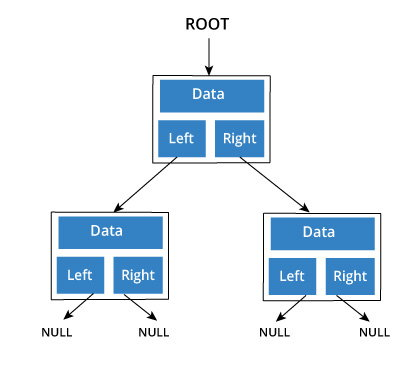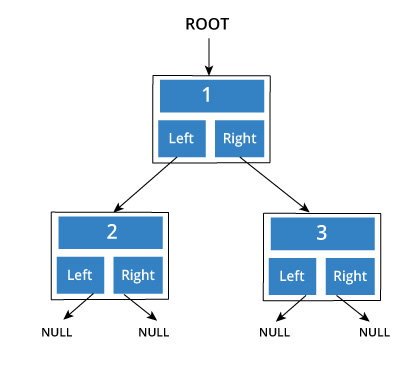# Tree Data Structure

A linked list is a chain of nodes connect through "next" pointers. A tree is similar, but each node can be connected to multiple nodes.

When we talk about tree, mostly we mean binary tree, that is a structure that has two children, left and right.

## Binary Tree Representation

A node of a binary tree is represented by a structure containing a data part and two pointers to other structures of the same type.

``````struct node
{
int data;
struct node *left;
struct node *right;
};```
```A special pointer called ROOT points to the node that is the parent of all the other nodes.

Also, the nodes that don't have any children have their left and right pointers point to `NULL`.

A basic binary tree with three nodes can be created as:

``````/* Initialize nodes */
struct node *root;
struct node *one = NULL;
struct node *two = NULL;
struct node *three = NULL;

/* Allocate memory */
one = malloc(sizeof(struct node));
two = malloc(sizeof(struct node));
three = malloc(sizeof(struct node));

/* Assign data values */
one->data = 1;
two->data = 2;
three->data = 3;

/* Connect nodes */
one->left = two;
one->right = three;
two->left = NULL;
two->right = NULL;
three->left = NULL;
three->right = NULL;

/* Save address of first node in root */
root = one;``````

In just a few steps, we have created a binary tree with three nodes.Trees can be much more deep and complex than this. The data stored in each node can be much more complex and there can be more children than just two.

## Applications of Trees

Trees and their variants are an extremely useful data structure with lots of practical applications.

• Binary Search Trees(BSTs) are used to quickly check whether an element is present in a set or not.
• Heap is a kind of tree that is used for heap sort.
• A modified version of tree called Tries is used in modern routers to store routing information.
• Most popular databases use B-Trees and T-Trees, which are variants of the tree structure we learned above to store their data
• Compilers use a syntax tree to validate the syntax of every program you write.

## Complete C program to implement Tree

``````#include <stdio.h>
#include <stdlib.h>

struct node {
int data;
struct node* left;
struct node* right;
};

struct node* createNode(value){
struct node* newNode = malloc(sizeof(struct node));
newNode->data = value;
newNode->left = NULL;
newNode->right = NULL;

return newNode;
}

struct node* insertLeft(struct node *root, int value) {
root->left = createNode(value);
return root->left;
}

struct node* insertRight(struct node *root, int value){
root->right = createNode(value);
return root->right;
}

int main(){
struct node *root = createNode(1);
insertLeft(root, 2);
insertRight(root, 3);

printf("The elements of tree are %d %d %d", root->data, root->left->data, root->right->data);
}``````

The output of the program will be

`1 2 3`